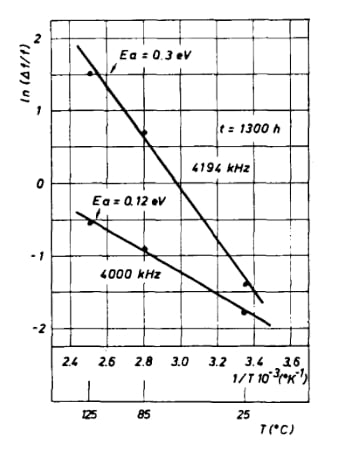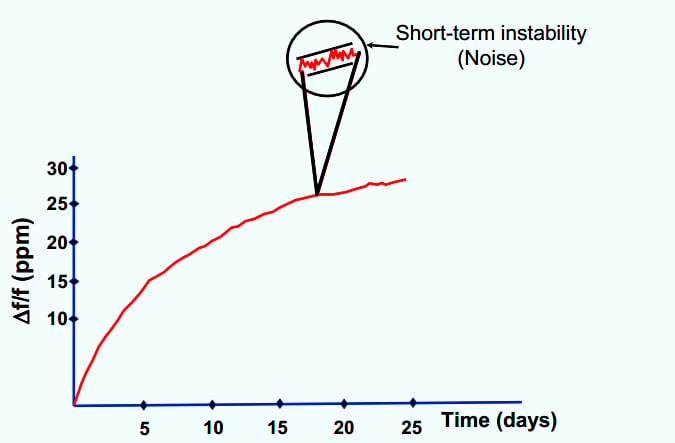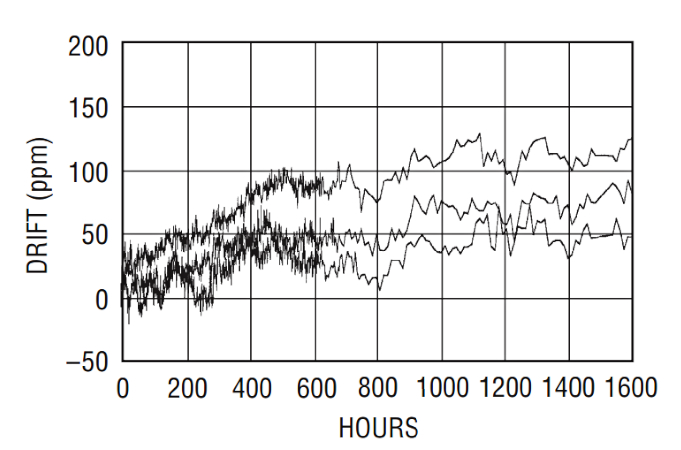Technical Article

# Using the Arrhenius Equation to Predict Electronic Component Aging

August 18, 2022 by Dr. Steve Arar

## Learn about calculating the activation energy of the aging process and some of the contradictory opinions about the Arrhenius equation's usefulness when predicting a crystal's aging process.

In a previous article, we discussed that the high temperature accelerated aging method is an efficient technique that allows the manufacturers to determine the long-term stability of the electronic components using relatively shorter test durations. For example, with this method, the data obtained from a 30-day test might be sufficient to determine the drift of a crystal after one year with acceptable accuracy. To apply this technique, we need to know the activation energy of the aging process.

### The Arrhenius Equation—Calculate the Activation Energy

The Arrhenius equation, given below, specifies the dependence of the rate constant of a chemical reaction on the absolute temperature:

$$Process \text{ } Rate\text{ }(PR) = Ae^{-\frac{E_a}{K_BT}}$$

##### Equation 1.

To apply this equation to the aging prediction problem, we need to know the activation energy of the aging process Ea. This parameter can be calculated from aging data at two or more temperatures. Theoretically, having aging data at two different temperatures, we can use Equation 1 to calculate Ea. However, with more data points, we can measure this parameter more accurately. For example, the paper “Aging Prediction of Quartz Crystal Units” gathers the aging data of the same crystal type at three different temperatures: 25 °C, 85 °C, and 125 °C.

In this case, five samples of the same crystal type are soaked at each temperature for a test duration of 1300 hours. With the mean frequency drift of each group known, the Arrhenius equation is used to determine the activation energy of the aging process. This study uses passive aging, meaning that the crystals are not powered during the bake, and measurements are made at room temperature before and after the bake. Figure 1 shows the results of this experiment for two different crystal types having frequencies of 4000 kHz and 4194 kHz.##### Figure 1. A chart showing the results of the experiment of two different crystal types. Image used courtesy of M.R. Miljkovic

The figure plots the natural logarithm of the variation in the crystal resonant frequency $$(ln(\frac{\Delta f}{f}))$$ against $$\frac{1}{T}$$.

Note that a straight line can be fit to these data points. This verifies that the aging process of the tested crystals obeys the Arrhenius equation and, thus, the temperature dependence of the aging process can be predicted by Equation 1.

#### Not All Crystals Have the Same Activation Energy!

The activation energy of the aging process obtained from different types of a given electronic component might not be the same. For example, as shown in Figure 1, the 4000 kHz and 4194 kHz crystals used in the aforementioned study have activation energies of 0.12 eV and 0.3 eV, respectively. In fact, the authors of this study compare the data from several different research papers and conclude that the activation energy of the aging of different crystal units can vary over a wide range from 0.08 eV to 0.65 eV.

Therefore, to use the Arrhenius equation, we need to first determine the activation energy of the crystal type under test. However, different samples from the same crystal type should have identical activation energies. For example, to obtain the Arrhenius plots in Figure 1, five samples of each crystal type are used. Then, having Ea and assuming that the aging process obeys the Arrhenius law, we can apply the equation presented in the previous article to predict the temperature dependence of the aging process with acceptable accuracy.

### Crystal Aging Tests: the Logarithmic Relationship of Aging With Time

The aging prediction method we have discussed in this two-part series is based on a relatively simple model. A significant amount of research is conducted to better understand the aging characteristics of crystals. These studies can help crystal manufacturers and researchers to more accurately predict crystal aging. However, there are accepted rules of thumb that are used by some crystal manufacturers to estimate the aging of one year at room temperature.

Military specifications have used accelerated aging tests at 85°C for 30 days and 105°C for 168 hours as the equivalent aging of one year at room temperature. The aging of one year at 25 °C is also estimated by a test at 85°C for 1000 hours. Different manufacturers might mention different test conditions in their documents. For example, IQD uses the general rule of 85°C for 30 days whereas Connor-Winfield uses 85°C for 1000 hours as its standard for qualification. Tests that are at a lower temperature over a longer period of time are expected to more accurately predict the aging effect.

Note that most crystal aging happens during the first year due to the logarithmic relationship of aging with time. Figure 2 shows a typical aging curve.##### Figure 2. An example of a typical aging curve. Image used courtesy of J. Vig

That’s why crystal datasheets usually specify the aging in the first year, e.g. a crystal might be specified to have aging of ±5 ppm in the first year.

Note that this crystal will NOT drift by ±25 ppm after 5 years because aging is not a linear function of time. As a rule of thumb, we can consider a maximum drift of ±10 ppm over 10 years due to crystal aging.

### The Arrhenius Formula and Aging Prediction—Useful Technique or Deliberate Lie?

There are some contradictory opinions about applying the Arrhenius equation to the aging prediction problem both in research papers and technical documents from manufacturers. For example, while Vishay considers the equation derived from the Arrhenius law a “widely accepted” method for resistor aging prediction and TI refers to the Arrhenius equation as “a simple but remarkably accurate formula." While Linear Technology calls it “a deliberate lie” in the context of aging prediction of voltage references.

#### Arrhenius Law Example Using the LT1461

According to the Linear Technology document, the typical 1000-hour long-term drift of the LT1461, which is a precision voltage reference, at 130 °C is 120 ppm. With activation energy of E= 0.7 eV, we can use Equation 1 to estimate the 1000-hour drift at 30 °C. Based on the Arrhenius law, the acceleration factor (AF) achieved by increasing the temperature is given by:

$$AF = \frac{PR(T_{test})}{PR(T_{use})} = e^{\frac{E_{a}}{K_{B}}\big(\frac{1}{T_{use}}-\frac{1}{T_{test}}\big)}$$

Where:

• Ttest = 130+273 = 403 K
• Tuse = 30+273 = 303 K
• KB = 8.617✕10-5 eV/K

Substituting these values, we obtain an acceleration factor of about 770. We expect the typical 1000-hour long-term drift of the LT1461 at 30 °C to be 120 ppm divided by 770, which is about 0.156 ppm. Figure 3 shows the measured long-term drift of the LT1461 at 30 °C.##### Figure 3. The LT1461 at 30 °C measured long-term drift. Image used courtesy of Linear Technology

From this experiment, the 1000-hour drift of the device is between about 35 ppm and 110 ppm. The typical 1000-hour long-term drift of the LT1461 at 30 °C is specified at 60 ppm by the company. These drift values are much greater than that predicted by the accelerated aging method (0.156 ppm). This shows that the aging process of the LT1461 doesn’t obey the Arrhenius law. In other words, if we gather the aging data at different temperatures and plot the natural logarithm of the drift against $$\frac{1}{T}$$, we won’t be able to fit a straight line to the data points unlike the case of the crystal data in Figure 1.

### The Arrhenius Equation for Age Prediction—Useful But Limited

This shows that the usefulness of the Arrhenius equation depends on the characteristics of the device under test. Although the aging data in Figure 1 obey the Arrhenius law, we cannot conclude that this equation can model the aging of other crystal types with acceptable accuracy. The Arrhenius equation can be a useful tool in certain cases; however, it has its own limitations. For example, it should be noted that the Arrhenius law characterizes the speed of a single chemical reaction. If several different mechanisms are significantly contributing to the overall aging effect of a device, the accuracy of the Arrhenius law can be affected.

In the next article, we’ll continue this discussion and take a look at the aging behavior of resistors, voltage references, and amplifiers.